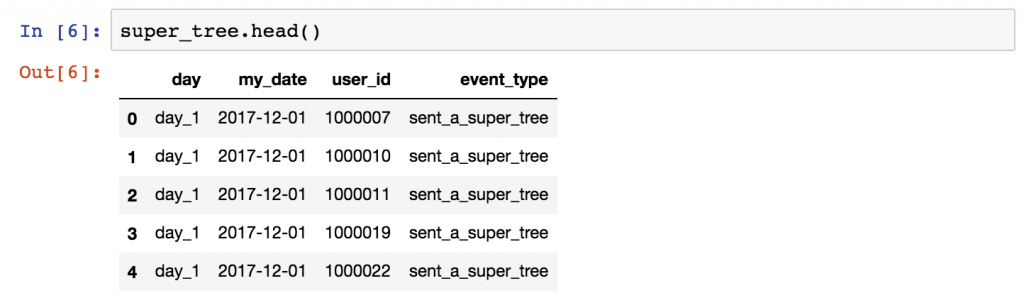# Top 5 Python Libraries You Must Know In 2021

Python is an ocean of libraries that serve various purposes and as a python developer, you must have to know about these libraries. Python Libraries You Must know:

## Numpy

Numpy will help you to manage multi-dimensional arrays very efficiently. Maybe you won’t do that directly, but since the concept is a crucial part of data science, many other libraries (well, almost all of them) are built on Numpy. Simply put: without Numpy you won’t be able to use Pandas, Matplotlib, Scipy or Scikit-Learn. That’s why you need it on the first hand.

But on the other hand, it also has a few well-implemented methods. I quite often use Numpy’s random function, which I found slightly better than the random module of the standard library. And when it comes to simple predictive analytics tasks like linear or polynomial regression, Numpy’s polyfit function is my favorite. (More about that in another article.)

## Pandas

To analyze data, we like to use two-dimensional tables – like in SQL and in Excel. Originally, Python didn’t have this feature. Weird, isn’t it? But that’s why Pandas is so important! I like to say, Pandas is the “SQL of Python.” (Eh, I can’t wait to see what I will get for this sentence in the comment section… ;-)) Okay, to be more precise: Pandas is the library that will help us to handle two-dimensional data tables in Python. In many senses it’s really similar to SQL, though.a pandas dataframe

With pandas, you can load your data into data frames, you can select columns, filter for specific values, group by values, run functions (sum, mean, median, min, max, etc.), merge dataframes and so on. You can also create multi-dimensional data-tables.

That’s a common misunderstanding, so let me clarify: Pandas is not a predictive analytics or machine learning library. It was created for data analysis, data cleaning, data handling and data discovery… By the way, these are the necessary steps before you run machine learning projects, and that’s why you will need pandas for every scientific project, too.

If you start with Python for Data Science and you learned the basics of Python, I recommend that you focus on learning Pandas next. These short article series of mine will help you: Pandas for Data Scientists.

## Matplotlib

I hope I don’t have to detail why data visualization is important. Data visualization helps you to better understand your data, discover things that you wouldn’t discover in raw format and communicate your findings more efficiently to others.
The best and most well-known Python data visualization library is Matplotlib. I wouldn’t say it’s easy to use… But usually if you save for yourself the 4 or 5 most commonly used code blocks for basic line charts and scatter plots, you can create your charts pretty fast.Here’s another article that introduces Matplotlib more in-depth: How to use matplotlib.

## Scikit-Learn

Without any doubt the fanciest things in Python are Machine Learning and Predictive Analytics. And the best library for that is Scikit-Learn, which simply defines itself as “Machine Learning in Python.” Scikit-Learn has several methods, basically covering everything you might need in the first few years of your data career: regression methods, classification methods, and clustering, as well as model validation and model selection. You can also use it for dimensionality reduction and feature extraction.

(Get started with my machine learning tutorials here: Linear Regression in Python using sklearn and numpy!)

a simple classification with a random forest model in Scikit Learn.

## Scipy

This is kind of confusing, but there is a Scipy library and there is a Scipy stack. Most of the libraries and packages I wrote about in this article are part of the Scipy stack (that is for scientific computing in Python). And one of these components is the Scipy library itself, which provides efficient solutions for numerical routines (the math stuff behind machine learning models). These are: integration, interpolation, optimization, etc.

Just like Numpy, you most probably won’t use Scipy itself, but the above-mentioned Scikit-Learn library highly relies on it. Scipy provides the core mathematical methods to do the complex machine learning processes in Scikit-learn. That’s why you have to know it.

## Conclusion

The five most essential Data Science libraries and packages are:

• Numpy
• Pandas
• Matplotlib
• Scikit-Learn
• Scipy

Get them, learn them, use them and they will open a lot of new doors in your data science career!